# Surface Area of Prisms and Cylinders Area The

• Slides: 16Surface Area of Prisms and Cylinders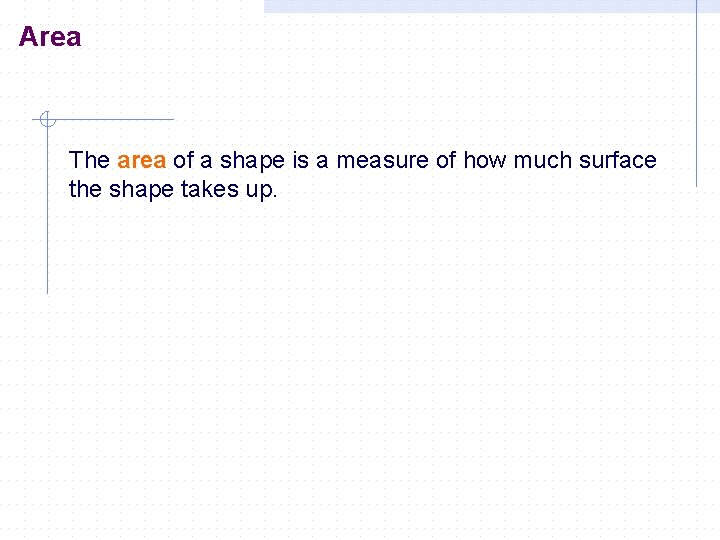Area The area of a shape is a measure of how much surface the shape takes up.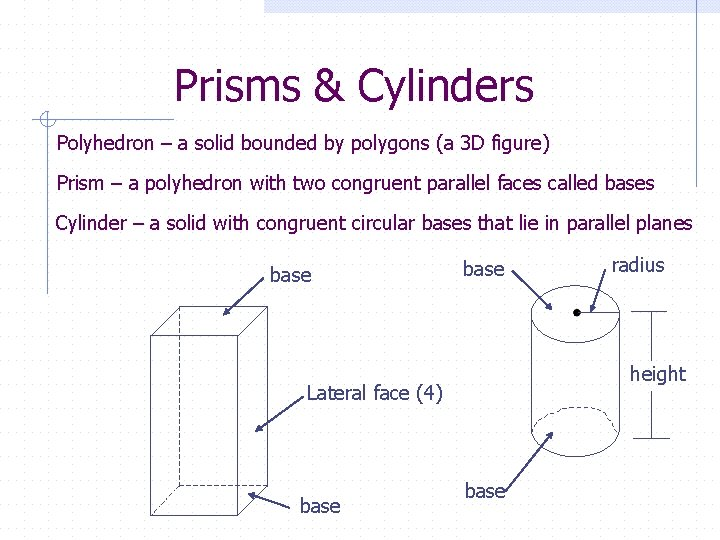Prisms & Cylinders Polyhedron – a solid bounded by polygons (a 3 D figure) Prism – a polyhedron with two congruent parallel faces called bases Cylinder – a solid with congruent circular bases that lie in parallel planes base height Lateral face (4) base radius basePrisms are classified by the shapes of their bases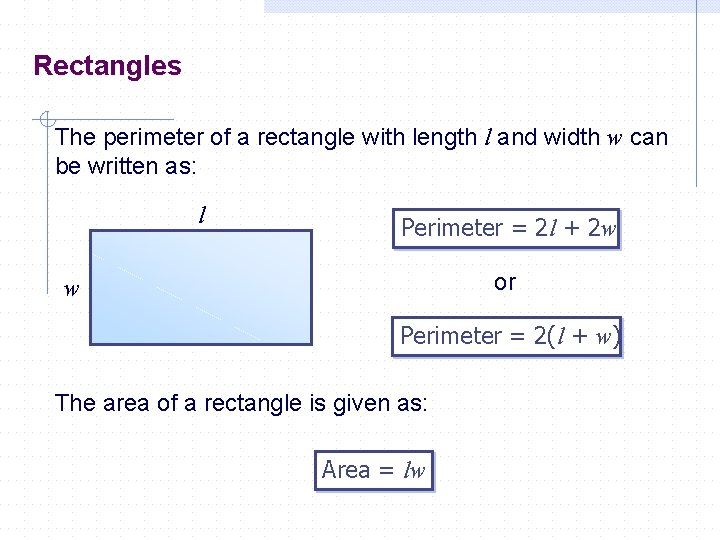Rectangles The perimeter of a rectangle with length l and width w can be written as: l Perimeter = 2 l + 2 w or w Perimeter = 2(l + w) The area of a rectangle is given as: Area = lw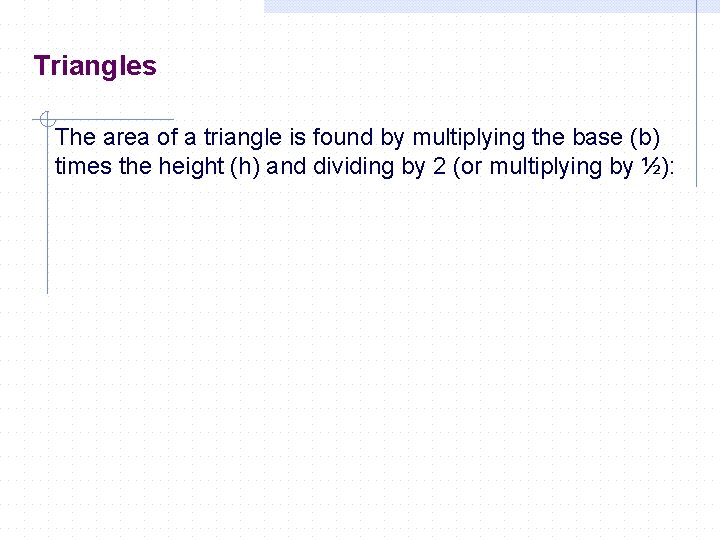Triangles The area of a triangle is found by multiplying the base (b) times the height (h) and dividing by 2 (or multiplying by ½):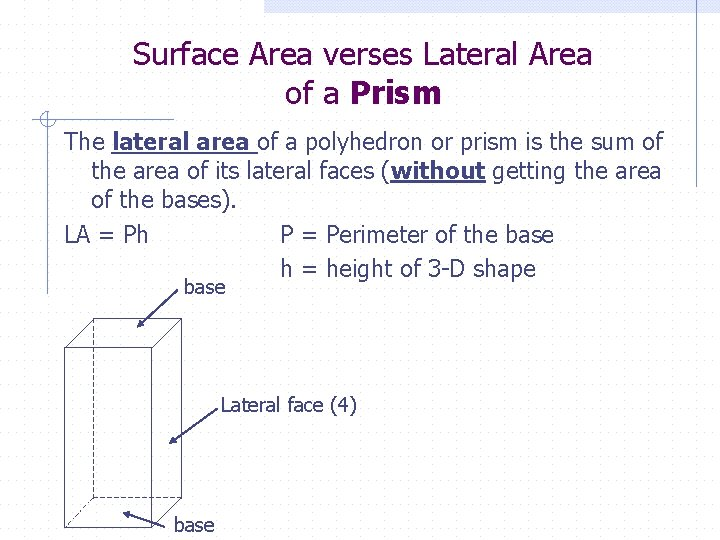Surface Area verses Lateral Area of a Prism The lateral area of a polyhedron or prism is the sum of the area of its lateral faces (without getting the area of the bases). LA = Ph P = Perimeter of the base h = height of 3 -D shape base Lateral face (4) base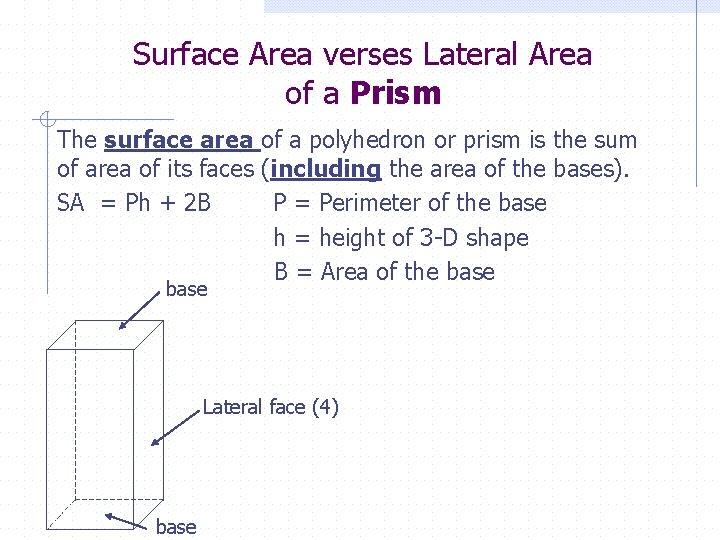Surface Area verses Lateral Area of a Prism The surface area of a polyhedron or prism is the sum of area of its faces (including the area of the bases). SA = Ph + 2 B P = Perimeter of the base h = height of 3 -D shape B = Area of the base Lateral face (4) base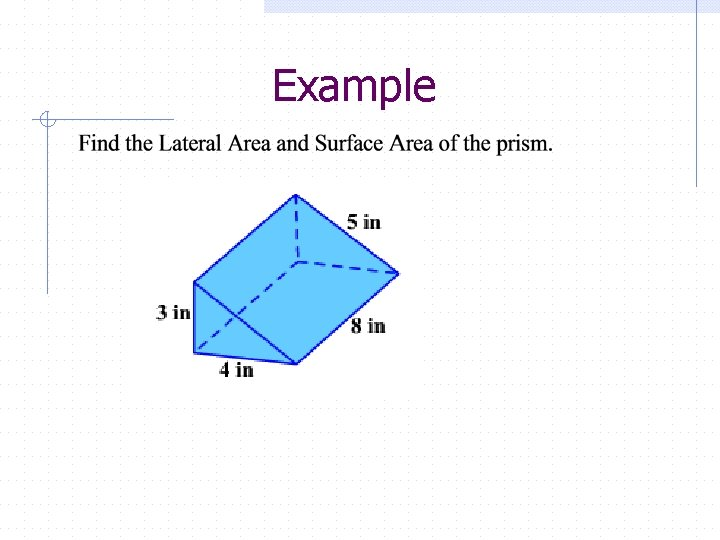Example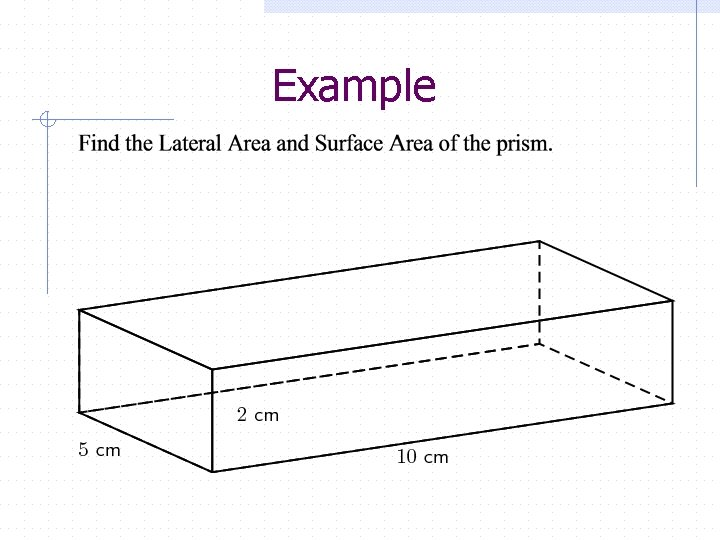ExampleThe circumference of a circle For any circle, The Circumference is found through the formula: C = πd Inversely, if you have the circumference, you can find the diameter through the formula: circumference diameter = π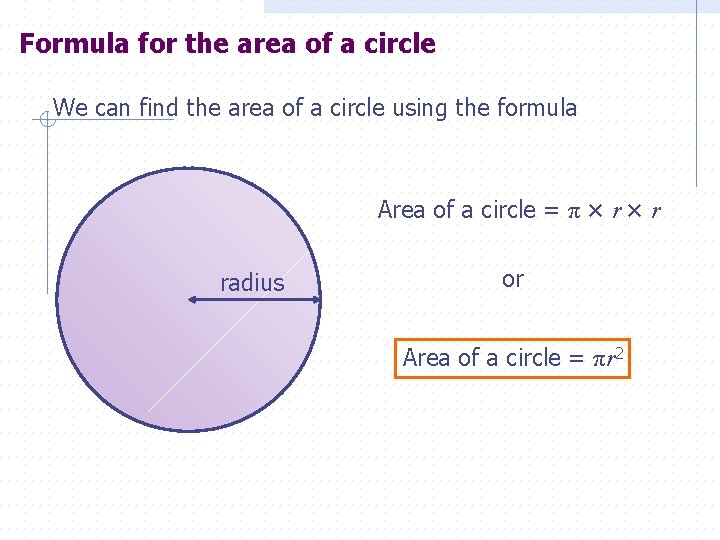Formula for the area of a circle We can find the area of a circle using the formula Area of a circle = π × r radius or Area of a circle = πr 2Surface Area verses Lateral Area of a Cylinder The lateral area of a cylinder is the area of its curved surface. LA = base radius height base r = radius of the base h = height of 3 -D shape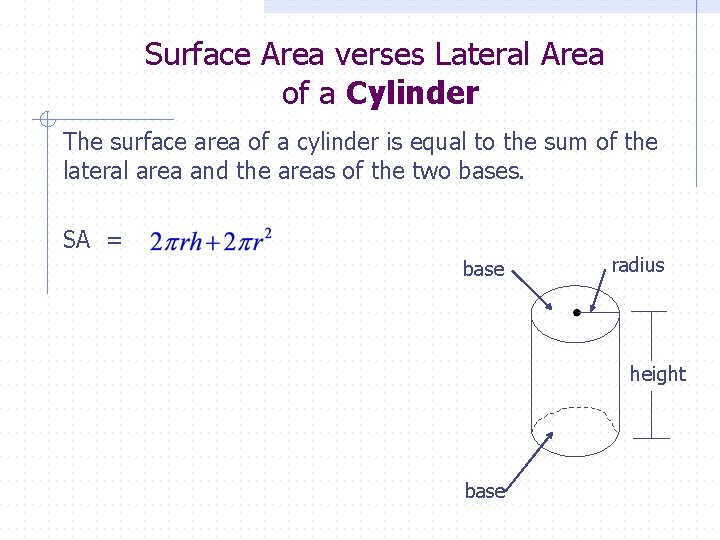Surface Area verses Lateral Area of a Cylinder The surface area of a cylinder is equal to the sum of the lateral area and the areas of the two bases. SA = base radius height baseScaling If we scale the dimensions of a prism or any other 3 D figure, we are multiplying each dimension (length, width, height, etc) by the scale factor. For example, if we have the following figure and scale it by 2, It’s new dimensions are 4 cm by 10 cm, by 20 cmHomework Page Page 16 -17, #6, 7 18, #11 A-C 22, #24 59, #8 -10 60, #11 61, #18 -20, 22, 23